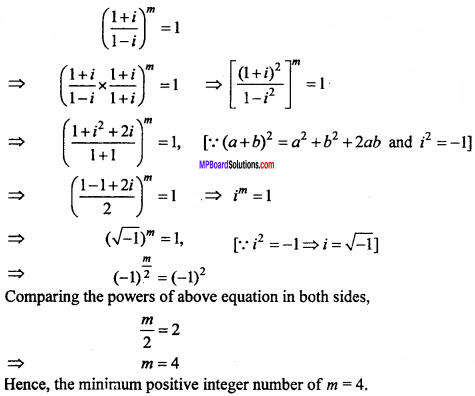# MP Board Class 11th Maths Important Questions Chapter 5 Complex Numbers and Quadratic Equations

## MP Board Class 11th Maths Important Questions Chapter 5 Complex Numbers and Quadratic Equations

### Relations and Functions Important Questions

Relations and Functions Objective Type Questions

(A) Choose the correct option :

Question 1.
If x + iy = 2 + 3i, then (x, y) will be :
(a) (3, 2)
(b) (2, 3)
(c) (- 2,- 3)
(d) (3, 3)
(b) (2, 3)

Question 2.
The simplest form of $$\frac { 1 + i }{ 1 – i }$$ :
(a) – i
(b) i
(c) ± i
(d) None of these.
(b) i

Question 3.
If z is a complex number, then z + z is :
(a) Real number
(b) Imaginary number
(c) Nothing can be said
(d) None of these.
(a) Real number

Question 4.
The value of $$\sqrt {i}$$ = ……………..
(a) ± $$\frac { 1 – i }{ \sqrt { 2 } }$$
(b) ± $$\frac { 1 + i }{ \sqrt { 2 } }$$
(c) ± (1 + i)
(d) ± (1 – i)
(b) ± $$\frac { 1 + i }{ \sqrt { 2 } }$$Question 5.
Number of solution of the equation z + z = 0 is :
(a) 1
(b) 2
(c) 3
(d) 4
(d) 4

Question 6.
Argument of complex number 0 is :
(a) 0
(b) π
(c) 2π
(d) Not defined
(d) Not defined

Question 7.
Conjugate of $$\frac { 1 – i }{ 1 + i }$$ is :
(a) $$\frac { 1 – i }{ 1 + i }$$
(b) $$\frac { 1 – i }{ 1 – i }$$
(c) i
(d) – i
(c) i

Question 8.
Argument (z1.z2) = ………………
(a) arg (z1) – arg (z2)
(b) arg (z1) + arg (z2)
(c) arg (z1). arg (z2)
(d) None of these
(b) arg (z1) + arg (z2)Question 9.
The least positive integer n for which $$\frac { 1 – i }{ 1 + i }$$n = 1 is :
(a) 2
(b) 4
(c) 8
(d) 12
(b) 4

Question 10.
If |z – $$\frac { 4}{ z}$$| = 2, then |z| has maximum value:
(a) $$\sqrt {3}$$ + 1
(b) $$\sqrt {5}$$ + 1
(c) 2
(d) 2 + $$\sqrt {2}$$
(b) $$\sqrt {5}$$ + 1

(B) Match the following :1. (d)
2. (a)
3. (f)
4. (b)
5. (c)
6. (e)

(c) Fill in the blanks :

1. The value of (1 + i)4 (1 + $$\frac {1}{i}$$)4 is ……………….
2. The value of $$\frac {1}{i}$$ is ……………….
3. The value of z – $$\bar { z }$$ is ……………….
4. Argument of – 1 – i is ……………….
5. polar form of i3 is ……………….
6. Argument of complex number is $$\frac { 1+\sqrt { 3i } }{ \sqrt { 3 } +i }$$ is ……………….
7. If |$$\frac { z – i }{ z + i }$$| = 1, then locus of z will be ……………….
8. If x + $$\frac {1}{x}$$ = 2cosθ, then the value of xn = $$\frac { 1 }{ { x }^{ n } }$$ will be ……………….
9. If z = 2 – 3i, then the value of z. $$\bar { z }$$ is ……………….
10. If 2 + (x + yi) = 3 – i, the x = ……………… and y = ……………….

1. 16
2. – i
3. Imaginary number
4. $$\frac {5π}{4}$$
5. cos$$\frac {π}{2}$$ – i sin$$\frac {π}{2}$$
6. $$\frac {π}{6}$$
7. X – axis
8. 2cos nθ
9. 13
10. 1, – 1

(D) Write true / false :

1. Additive inverse of complex number – 2 + 5i is 2 – 5 i.
2. If | z2 – 1 |= z2 + 1, then z lies on a circle.
3. If | z1 | = 12 and | z2 – 3 – 4i | = 5, then the least value of | z1 – z2 | is 7.
4. If arg(z) < 0, then arg(- z) – arg(z) = π.
5. Polar form of 1 + $$\sqrt {- 1}$$ is $$\sqrt {2}$$(cos$$\frac {π}{4}$$ + isin$$\frac {π}{4}$$).
6. If z is a complex number such that | z | ≥ 2, then the minimum value of |z + $$\frac { 1 }{ 2 }$$ | equal $$\frac { 5 }{ 2 }$$

1. True
2. False
3. False
4. True
5. True
6. False.

(E) Write answer in one word / sentence :

1. If Z1 = 2 – i, z2 = 1 + i, them find the value $$\frac { { { z }_{ 1 }+{ z }_{ 2 }+1 } }{ { z }_{ 1 }-{ z }_{ 2 }+1 }$$
2. Modulus of $$\frac {1 – i }{ 1 + i }$$ – $$\frac { 1 – i }{ 1 + i }$$ will be.
3. Polar form of complex number $$\sqrt {3}$$ + i will be.
4. If Z1 = 2 – i and z2 = – 2 + i, then Re($$\frac { { { z }_{ 1 }{ z }_{ 2 } } }{ { z }_{ 1 } }$$) will be
5. If the number $$\frac { z – 1 }{ z + 1 }$$ is purly imaginary, then the value of |z| will be.

1. $$\sqrt {2}$$
2. 2
3. 2(cos$$\frac {π}{6}$$ + isin$$\frac {π}{6}$$)
4. $$\frac { – 2 }{ 5 }$$
5. 1

Question 1.
If 1, ω, ω2 are the cube root of unity then find the value of ω3n.
Solution:
Since ω3 = 1
ω3n = (ω3)n = (1)n = 1.

Question 2.
Find the value of i4n.
Solution:
i4n = (i4)n = (i2.i2)n = [(- 1)(- 1)]n = (1)n = 1.

Question 3.
Find the condition of two complex numbers x + iy and a + ib are compaired.
Solution:
If x + iy = a + ib
Then, x = a, y = b.

Question 4.
Write conjugate of a complex number z = a – ib.
Solution:
z = conjugate of a – ib = a – (- ib)
= a + ib.Question 5.
Write the complex number – 63 in ordered form.
Solution:
Ordered form of z = a + ib is (a, b).
Given: z = 0 – 6i
Ordered pair (0, – 6).

Question 6.
Write the complex number 3 – $$\sqrt { 7i }$$ in ordered form.
Solution:
Ordered pair of z = a + ib is (a, b).
∴Ordered pair of 3 – $$\sqrt { 7i }$$ = (3, – $$\sqrt { 7i }$$)

Question 7.
Find the value of i + $$\frac { 1 }{ i }$$.
Solution:
i + $$\frac { 1 }{ i }$$ = $$\frac { { i }^{ 2 }+1 }{ i }$$ = $$\frac { – 1 + i }{ i }$$ = 0.

Question 8.
Find the value of i-19.
Solution:
i-19 = $$\frac { 1 }{ { i }^{ 19 } }$$ = $$\frac { 1 }{ { i }^{ 18 }i }$$ = $$\frac { 1 }{ (i^{ 2 })^{ 9 }.i }$$
= $$\frac { 1 }{ -1.i }$$ = $$\frac { – 1 }{ i }$$
= $$\frac { – 1.i }{ { i }^{ 2 } }$$ = i.

Question 9.
Write (1 – i)4 in form of a + ib.
Solution:
(1 – i)4 = [ (1 – i)2 ]2 (NCERT)
= [1 + i2 – 2i]2 = [1 – 1 – 2i]2, [∵ i2 = – 1]
= (- 2i)2 = 4i2 = 4(- 1) = – 4
= – 4 + 0i.

Question 10.
Find the modulus of complex number z = – 1 – i $$\sqrt {3}$$ .
Solution:
Z = – 1 – i$$\sqrt {3}$$
a = – 1, b = $$\sqrt {3}$$
Modulus = $$\sqrt { { a }^{ 2 }+{ b }^{ 2 } }$$ = $$\sqrt {1 + 3}$$ = $$\sqrt {2}$$

Question 11.
Find the modulus of complex number 1 – i.
Solution:
z = 1 – i
a = 1, b = – 1
Modulus = $$\sqrt { { a }^{ 2 }+{ b }^{ 2 } }$$ = $$\sqrt {1 + 1}$$ = $$\sqrt {2}$$

Question 12.
Find the sum of 2 – 3i and its conjugate.
Solution:
z = 2 – 3i and $$\bar { z }$$ = 2 + 3i
z + $$\bar { z }$$ = 2 – 3i + 2 + 3i = 4.

Question 13.
Solve the quadratic equation x2 + 2 = 0. (NCERT)
Solution:
x2 + 2 = 0
⇒ x2 = – 2
⇒ x2 = – 1 x 2
= 2i2 [∵ i2 = – 1]
x = ± $$\sqrt {2i}$$ or + $$\sqrt {2}$$i, – $$\sqrt {2}$$i

Question 14.
Solve the quadratic equation x2 + 3 = 0. (NCERT)
Solution:
x2 + 3 = 0
⇒ x2 = – 3 = – 1 x 3
⇒ x2 = 3i2, [∵ i2 = – 1]
x2 = ± $$\sqrt {3i}$$ or + $$\sqrt {3}$$i, – $$\sqrt {3}$$i

Question 15.
Write the triangle inequality for the two complex number Z1 and z2.
Solution:
| Z1| and | z2| are the two sides of any triangle
Then, | z0 + z2| ≤ | z1| +| z2|Question 16.
If T and 9 are the modulus and argument of the complex number a + ib, then write its polar form.
Solution:
Polar form of a + ib is r(cosθ + i sinθ).

Question 17.
If z = cosθ + i sinθ where |z| = 1, then find $$\frac { 1 }{ z }$$.
Solution:
$$\frac { 1 }{ z }$$ = cosθ – isinθ.

Question 18.
Write the statement of De – moivre’s theorem.
Solution:
If polar form of z = a + ib is r(cosθ + isinθ), then De – moivre’s theorem is
(cosθ + isinθ)n = cos nθ + i sin nθ.

Question 19.
Write polar form of i.
Solution:
a + ib = 0 + i. 1
r = $$\sqrt { { a }^{ 2 }+{ b }^{ 2 } }$$ =1, θ = tan-1$$\frac { b }{ a}$$ = tan-1∞ = $$\frac { π }{ 2}$$
Polar form of i = (cos$$\frac { π }{ 2}$$ + i sin $$\frac { π }{ 2}$$).

Question 20.
Find the polar form of (- 2 + 2i).
Solution:Question 1.
Convert ($$\frac { 1 }{ 3}$$ + 3i)3 in form of a + ib. (NCERT)
Solution: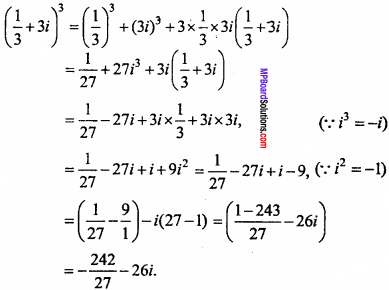Question 2.
Write ( – 2 – $$\frac { 1 }{ 3}$$i)3 in form of a + ib. (NCERT)
Solution:Question 3.
Write (5 – 3i)3 in the form of a + ib.
Solution:
(5 – 3i)3 = (5)3 – (3i)3 – 3(52.3i) + 3.5(3i)2
= 125 – 27.i3 – 75.3i + 15.9i2
= 125 – 27i.i2 – 225i + 15(- 9)
= 125 + 27i – 225i – 135
= – 10 – 198i.

Find the multiplicative inverse of following complex number :

Question 4.
4 – 3i
Solution:
Let the multiplicative inverse of 4 – 3i is a + ib.
Then, (4 – 3i) x (a + ib) = 1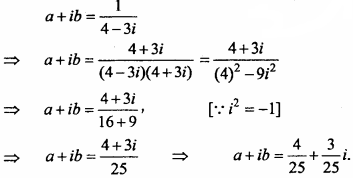Question 5.
$$\sqrt {5}$$ + 3i
Solution:
Let z = $$\sqrt {5}$$ + 3i
Be multiplicative inverseQuestion 6.
Find the polar form of the following :
(i) $$\frac { 1 + 7i }{ { 2 – i }^{ 2 } }$$, (ii) $$\frac { 1 + 3i }{ 1 – 2i }$$
Solution: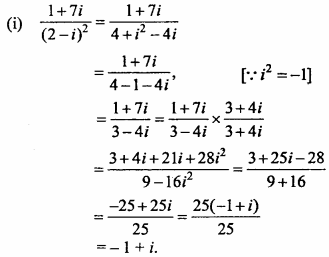Now, let – 1 + i = r(cosθ + i sinθ),
Then, r cosθ = – 1 …. (1)
and r sinθ = 1 …. (2)
Squaring and adding equation (1) and (2),
r2 = 1 + 1 = 2
⇒ r = $$\sqrt {2}$$
Put value of r in equation (1) and (2),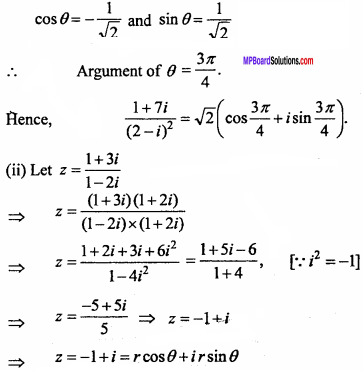Comparing the real and imaginary parts,
r cosθ = – 1
r sinθ = 1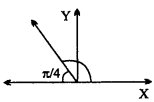Squaring and adding equation (1) and (2),∵ Real part is negative and imaginary part is positive of z, hence θ will lie in 4th quadrantQuestion 7.
Find modulus and argument of complex number z = – 1 – i$$\sqrt {3}$$ (NCERT)
Solution:
Let z = – 1 – i$$\sqrt {3}$$ = rcosθ + ir sinθComparing the real and imaginary parts,
rcosθ = – 1 …. (1)
rsinθ = – $$\sqrt {3}$$ …. (2)
Squaring and adding equation (1) and (2),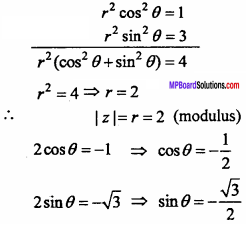Since both the real and imaginary part of z are negative.
∴ θ lies in third quadrant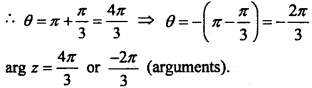Question 8.
Find the modulus and argument of z = – $$\sqrt {3}$$+ i.
Solution:
Let z = – $$\sqrt {3}$$+ i = rcosθ + ir sinθ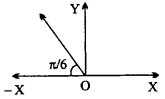Comparing the real and imaginary parts,
r cosθ = – $$\sqrt {3}$$
rsinθ = 1
Squaring and adding eqns. (1) and (2),
rcosθ = – $$\sqrt {3}$$ …. (1)
rsinθ = 1 …. (2)
Squaring and adding equation (1) and (2),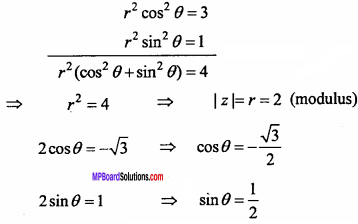Since both the real and imaginary part of z are negative and positive respectively.
∴ θ lies in 22nd quadrantQuestion 9.
Find the modulus and argument of 1 – i.
Solution:
Let z = 1 – i = rcosθ + ir smθ
Comparing the real and imaginary parts,
1 = r cosθ
and – 1 = rsinθ
Squaring and adding equation (1) and (2),
(1)2 + (- 1)2 = r2 cos20 + r2 sin20
⇒ r2(cos20 + sin20) = 1 + 1,
⇒ r2= 2 ⇒ r = $$\sqrt {2}$$ (modulus)
Dividing equation (2) by equation (1),Question 10.
Find square root of complex number 3 + 4i.
Solution:
Let $$\sqrt {3 + 4i}$$ = x + iy
On squaring,
3 + 4 i = (x + iy)2
⇒ 3 + 4i = x2 + 2ixy + i2y2
⇒ 3 + 4i = x2 + 2ixy – y2
⇒ 3 + 4i = (x2 – y2) + i(2xy)
x2 – y2 = 3 …. (1)
2xy = 4 …. (2)
(x2 + y2)2 = (x2 – y2)2 + 4x2y2
= (3)2 + (4)2
= 25
∴ x2 + y2 = 5 …. (3)
Now adding eqns. (1) and (3),
2x2 = 8
⇒ x2 = 4
⇒ x = ± 2
From equation (2), 2(± 2)y = 4
∴ y = ± 1
$$\sqrt { x + iy }$$ = ± 2 ± i.

Question 11.
Find square root of complex number 8 – 6i.
Solution:
Let $$\sqrt { 8 – 6i }$$ = x + iy
On squaring,
8 – 6i = (x + iy)2
⇒ 8 – 6i = x2 + 2ixy + i2y2 .
⇒ 8 – 6i = (x2 – y2) + 2ixy
∴ 8 = x2 – y2 …. (1)
and – 6 = 2 xy …. (2)
(x2 + y2)2 = (x2 – y2)2 + 4x2y2
(x2+y2)2 = (8)2 + (- 6)2
= 64 + 36 = 100
∴ x2 + y2 = 10 …. (3)
Now adding equation (1) and (3),
2x2 = 18
⇒ x2 = 9
⇒ x = ±3
From equation (3), y2 = 10 – 9 = 1
∴ y = ± 1
$$\sqrt { 8 – 6i}$$ = ± 3 ± i.

Question 12.
x2 + 3x + 9 = 0.
Solution:
Given : x2 + 3x + 9 = 0
Comparing the above equation by ax2 + bx + c = 0,
a = 1, b = 3, c = 9
Now, D = b2 – 4ac
= 32 – 4 x 1 x 9 = 9 – 36 = – 27 < 0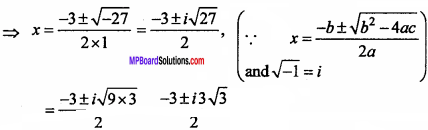Question 13.
– x2 + x – 2 = 0.
Solution:
Given : – x2 + x – 2 = 0
Comparing the above equation by ax2 + bx + c = 0,Question 14.
x2 + 3x + 5 = 0.
Solution:
Given : x2 + 3x + 5 = 0
Comparing the above equation by ax2 + bx+c = 0,
a = 1, b = 3, c = 5Question 15.
x2 – x + 2 = 0
Solution:
Given : x2 – x + 2 = 0
Comparing the above equation by ax2 + bx + c = 0,
a = 1, b = – 1, c = 2
Now, D = b2 – 4ac
= (- 1)2 – 4 x 1 x 2 = 1 – 8 = – 7 < 0Question 16.
If ($$\frac { 1 + i }{ 1 – i }$$) = 1, then And the minimum positive integer number of m. (NCERT)
Solution: